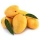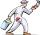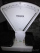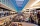# Fraction calculator

The calculator performs basic and advanced operations with fractions, expressions with fractions combined with integers, decimals, and mixed numbers. It also shows detailed step-by-step information about the fraction calculation procedure. Solve problems with two, three, or more fractions and numbers in one expression.

## Result:

### 21/5 + 13/4 = 79/20 = 3 19/20 = 3.95

Spelled result in words is seventy-nine twentieths (or three and nineteen twentieths).

### How do you solve fractions step by step?

1. Conversion a mixed number 2 1/5 to a improper fraction: 2 1/5 = 2 1/5 = 2 · 5 + 1/5 = 10 + 1/5 = 11/5

To find a new numerator:
a) Multiply the whole number 2 by the denominator 5. Whole number 2 equally 2 * 5/5 = 10/5
b) Add the answer from previous step 10 to the numerator 1. New numerator is 10 + 1 = 11
c) Write a previous answer (new numerator 11) over the denominator 5.

Two and one fifth is eleven fifths
2. Conversion a mixed number 1 3/4 to a improper fraction: 1 3/4 = 1 3/4 = 1 · 4 + 3/4 = 4 + 3/4 = 7/4

To find a new numerator:
a) Multiply the whole number 1 by the denominator 4. Whole number 1 equally 1 * 4/4 = 4/4
b) Add the answer from previous step 4 to the numerator 3. New numerator is 4 + 3 = 7
c) Write a previous answer (new numerator 7) over the denominator 4.

One and three quarters is seven quarters
3. Add: 11/5 + 7/4 = 11 · 4/5 · 4 + 7 · 5/4 · 5 = 44/20 + 35/20 = 44 + 35/20 = 79/20
For adding, subtracting, and comparing fractions, it is suitable to adjust both fractions to a common (equal, identical) denominator. The common denominator you can calculate as the least common multiple of both denominators - LCM(5, 4) = 20. In practice, it is enough to find the common denominator (not necessarily the lowest) by multiplying the denominators: 5 × 4 = 20. In the following intermediate step, the fraction result cannot be further simplified by canceling.
In other words - eleven fifths plus seven quarters = seventy-nine twentieths.

#### Rules for expressions with fractions:

Fractions - simply use a forward slash between the numerator and denominator, i.e., for five-hundredths, enter 5/100. If you are using mixed numbers, be sure to leave a single space between the whole and fraction part.
The slash separates the numerator (number above a fraction line) and denominator (number below).

Mixed numerals (mixed fractions or mixed numbers) write as integer separated by one space and fraction i.e., 1 2/3 (having the same sign). An example of a negative mixed fraction: -5 1/2.
Because slash is both signs for fraction line and division, we recommended use colon (:) as the operator of division fractions i.e., 1/2 : 3.

Decimals (decimal numbers) enter with a decimal point . and they are automatically converted to fractions - i.e. 1.45.

The colon : and slash / is the symbol of division. Can be used to divide mixed numbers 1 2/3 : 4 3/8 or can be used for write complex fractions i.e. 1/2 : 1/3.
An asterisk * or × is the symbol for multiplication.
Plus + is addition, minus sign - is subtraction and ()[] is mathematical parentheses.
The exponentiation/power symbol is ^ - for example: (7/8-4/5)^2 = (7/8-4/5)2

#### Examples:

subtracting fractions: 2/3 - 1/2
multiplying fractions: 7/8 * 3/9
dividing Fractions: 1/2 : 3/4
exponentiation of fraction: 3/5^3
fractional exponents: 16 ^ 1/2
adding fractions and mixed numbers: 8/5 + 6 2/7
dividing integer and fraction: 5 ÷ 1/2
complex fractions: 5/8 : 2 2/3
decimal to fraction: 0.625
Fraction to Decimal: 1/4
Fraction to Percent: 1/8 %
comparing fractions: 1/4 2/3
multiplying a fraction by a whole number: 6 * 3/4
square root of a fraction: sqrt(1/16)
reducing or simplifying the fraction (simplification) - dividing the numerator and denominator of a fraction by the same non-zero number - equivalent fraction: 4/22
expression with brackets: 1/3 * (1/2 - 3 3/8)
compound fraction: 3/4 of 5/7
fractions multiple: 2/3 of 3/5
divide to find the quotient: 3/5 ÷ 2/3

The calculator follows well-known rules for order of operations. The most common mnemonics for remembering this order of operations are:
PEMDAS - Parentheses, Exponents, Multiplication, Division, Addition, Subtraction.
BEDMAS - Brackets, Exponents, Division, Multiplication, Addition, Subtraction
BODMAS - Brackets, Of or Order, Division, Multiplication, Addition, Subtraction.
GEMDAS - Grouping Symbols - brackets (){}, Exponents, Multiplication, Division, Addition, Subtraction.
Be careful, always do multiplication and division before addition and subtraction. Some operators (+ and -) and (* and /) has the same priority and then must evaluate from left to right.

## Fractions in word problems:What is the sum of 2/3 and 3/10?A basket contains three types of fruits weighing 87/4 kg in all. If 23/4 kilograms of these are oranges, 48/7 kg are mangoes, and the rest are apples. What is the weight of the apples in the basket?
• Product and sumWhat is the product of two fourths  and the sum of three halves and four?
• Series and sequencesFind a fraction equivalent to the recurring decimal? 0.435643564356
• Math homeworkIt took Jose two-thirds of an hour to complete his math homework on Monday, three-fourths of an hour on Tuesday, any two- fifths of an hour on Wednesday. How many hours did it take Jose to complete his homework altogether?
• Mixing coloursLast summer, Mang Tinoy repainted his car. He mixed 2 3/8 cans of white paint, 1 1/3 cans of red paint, and 1 2/4 cans of blue paint. How much paint did he used overall?
• SavingsEva borrowed 1/3 of her savings to her brother, 1/2 of savings spent in the store and 7 euros left. How much did she save?
• SayavongSayavong is making cookies for the class. He has a recipe that calls for 3 and 1/2 cups of flour. He has 7/8 of a cup of wheat flour, and 2 and 1/2 cups of white flour. Does Mr. Sayavong have enough flour to make the cookies?
• The cost 2The cost of 5 apples is \$3.45, and 5 oranges are \$1.23. If Rachel buys one apple and one orange, then how much must she pay?
• Jo walksJo walks 3/4 of km to a friends home, 1/2 km to mall, and 2/3 km home. What total distance that joy covers?
• Sum of fractionsWhat is the sum of 2/3+3/5?Mike buys flowers to plant around his trees. 3/8 of the flowers are red. 1/3 of the flowers are pink. The rest of the flowers are white. Find the fraction of flowers that are white.What is the sign of expression: minus 18 start fraction 9 divided by 17 end fraction plus left parenthesis minus 18 start fraction 9 divided by 17 end fraction right parenthesis?